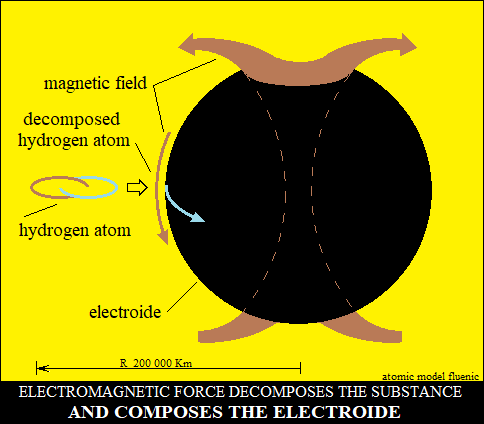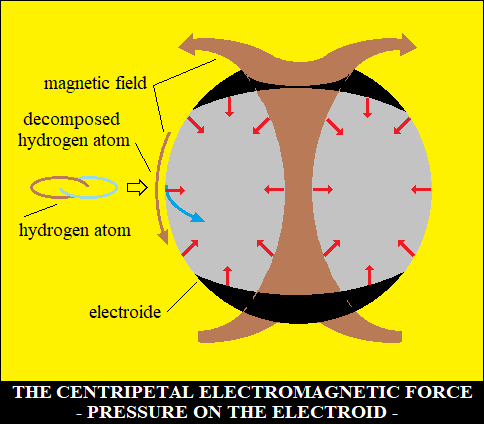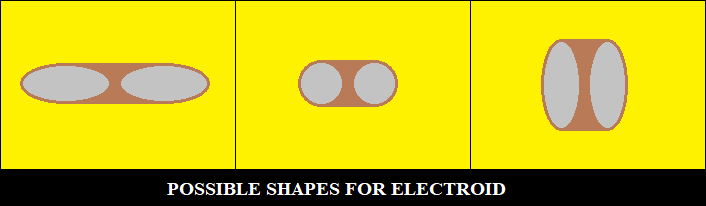How to form the sun electroidul
The electromagnetism of the sun is "built" by the centripetal EM force.
This force accelerates the substance centripetally,
generating increasing a EM pressure toward the center.
Where this pressure exceeds that of the hydrogen atom (double torus oscillator),
the EM circuits of the atom are opened and oriented by the EM force, stopping the oscillations.
The EM force and high EM pressure, organize the characteristic structure to the energy
with vector properties, a gigantic double torus, consisting of electroid and its magnetism.
EM pressure also increases in this structure, giving the electroide a sphere shape drilled
by the magnetic circuit and for the sun a unitary structure.
This structure is also detected in the center of the earth - the "solid core"
The kinetic energy (the centripetal EM force) and the potential energy (the electroide)
are the source of energy, one for the other, continuously.
The electroid shape is the ratio of the centripetal and angular EM accelerations.
Centripetal acceleration produces pressure - the electroid.
Angular acceleration produces centrifugal force - the shape of the electroid.<>